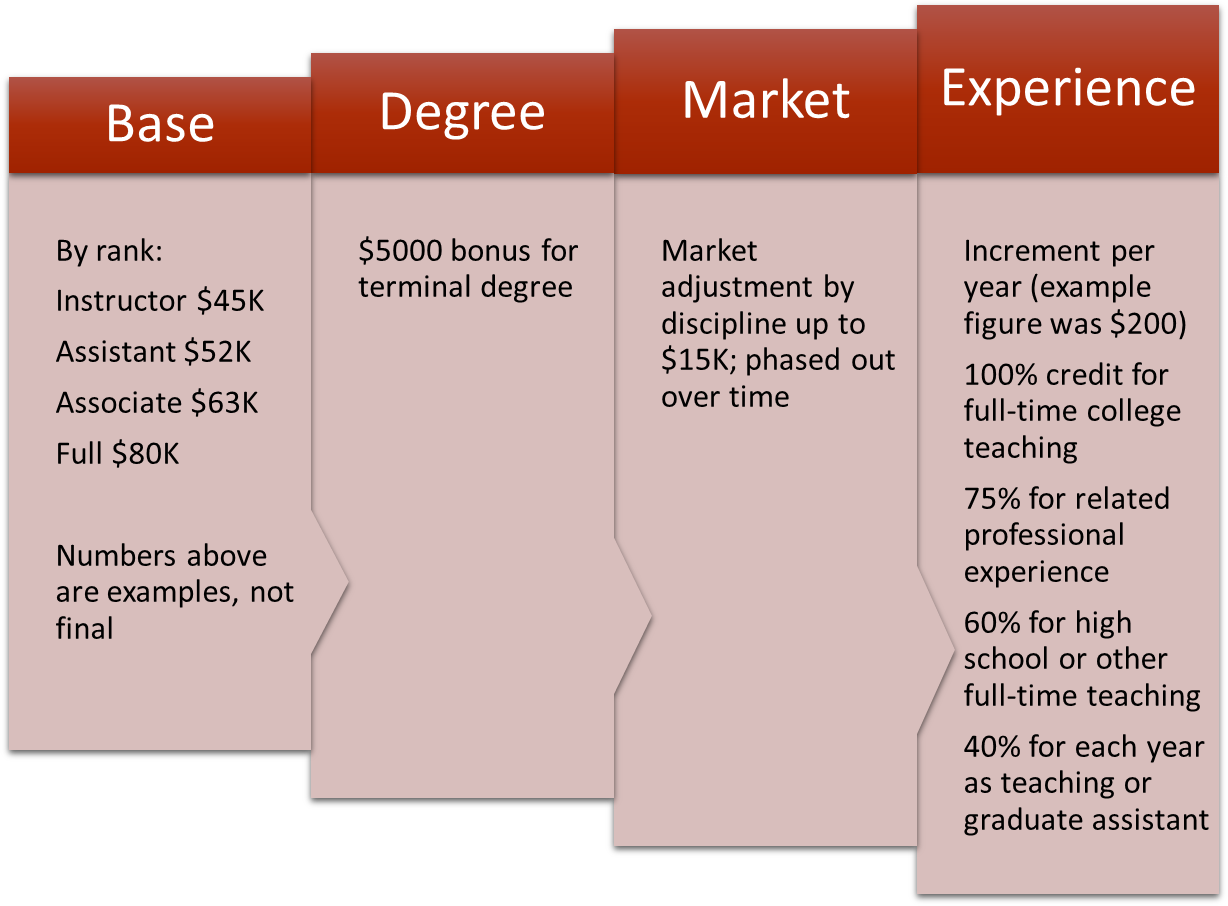# The Formula Forum

The current proposed model for the formula for salary targets is as follows:If you would like to calculate a target salary for your position or for a hypothetical position, you can use the formula calculator here:

## Formula calculator

Note: The calculator and the numbers above are based on rough example figures from the proposal and are not likely to be the final version of the formula.